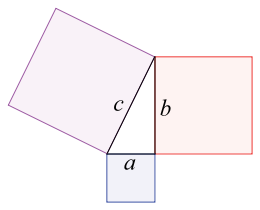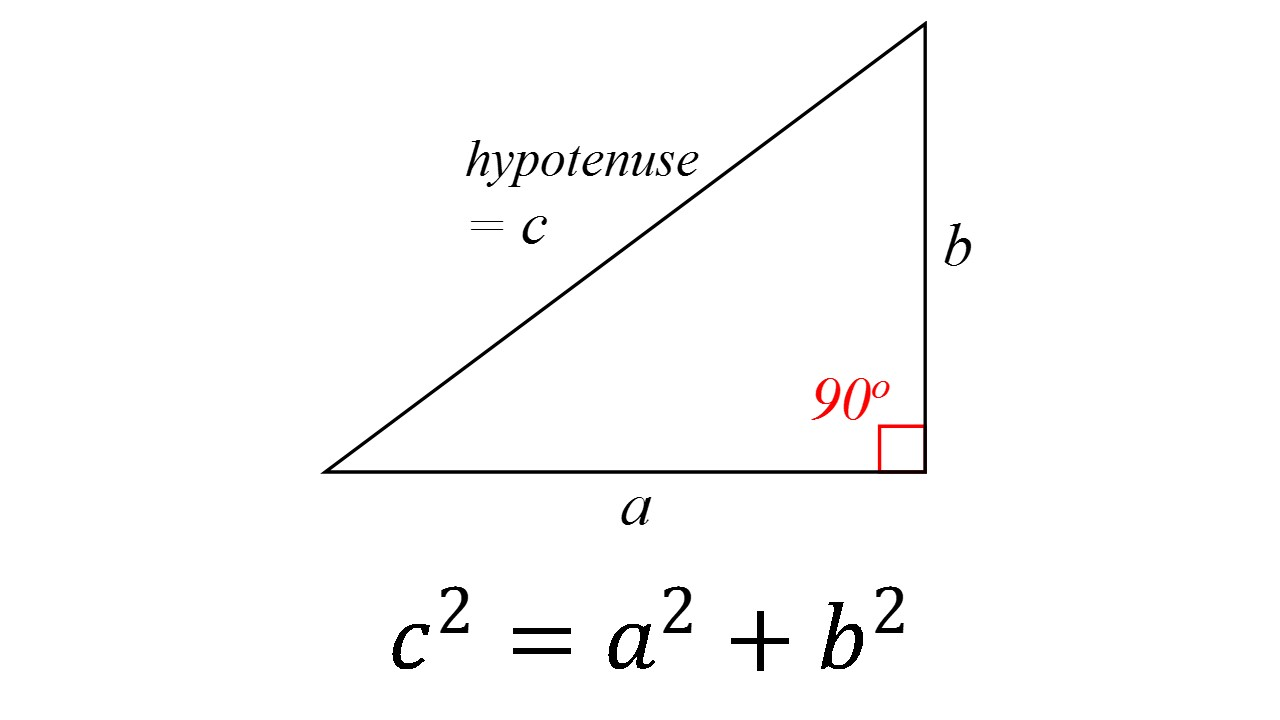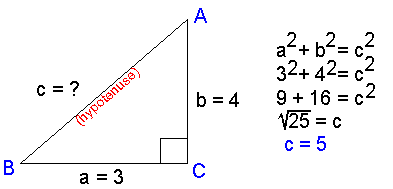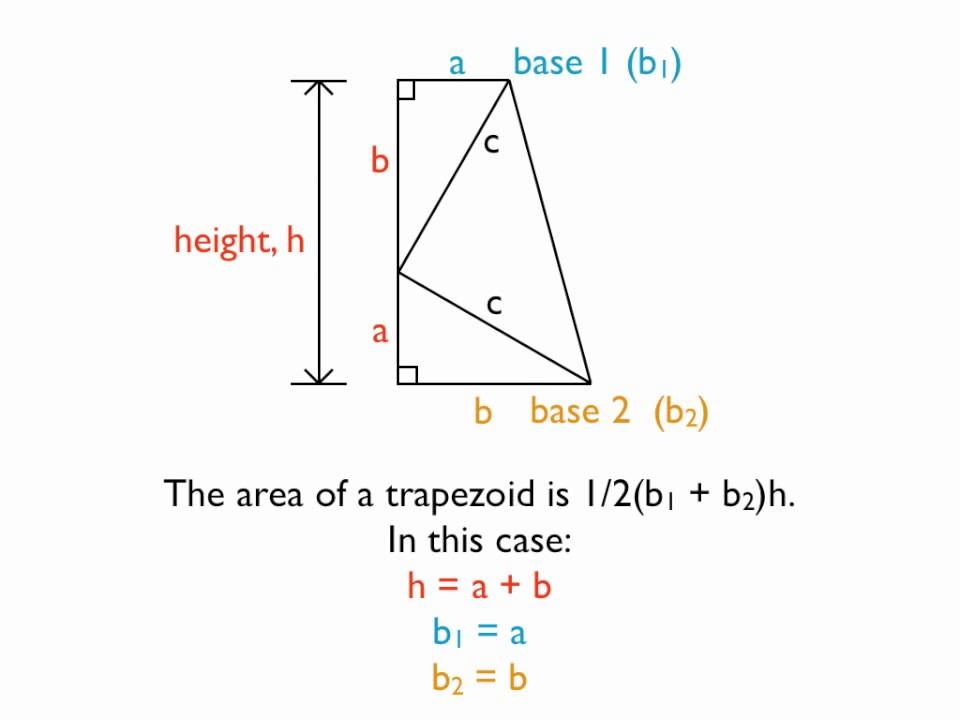# The Pythagorean Theorem And Its Evidence

This is a very Complex Topic and will take a good explanation and examples to prove the Formula,The Evidence of Your work, and the Evidence of Garfield's Evidence

• The definition of the Pythagorean Theorem - The Pythagorean theorem, also known as Pythagoras's theorem, is a fundamental relation in Euclidean geometry among the three sides of a right triangle. It states that the square of the hypotenuse (the side opposite the right angle) is equal to the sum of the squares of the other two sides.Now If the photo U see a(n) "a, b, and c" Those represent the side's The a (and) the b represent the shortest length of the triangle. And C would represent the longest side called the hypotenuseHere's some Information that will help you though your journey through Math.

-The Formula of The Pythagorean Theorem is a^2+b^2=c^2 As shown In the bottom of the 2nd picture.

• The Triangle MUST I repeat MUST be a right triangle and only a right triangle doesn't matter how it look or is drawn as long as It is a right triangle you will be good!

Now here's a example (problem) of the theorem• In the picture shown above it show a right triangle and its measurements "a and b" But not "c". Given the 2 measurements a=3 and b=4 We can solve for c.
• Now we will square 3 and 4. Which will leave: 9 and 16 Another thing We cant leave the answer 25. Here's a tip of knowledge To get the final answer, We will have to square root 25 and c^2 to get c by itself
• After square root 25 and c^2 we get c=5

• Now Before I finish, I will discuss (a little bit) about A famous Mathematics that change the triangle world FOREVER

James Garfield!!!!!

• In the picture below It show's the work involved in The evidence of Garfield• Now I have discuss all that can I can do and help I will make more Discuss Topic Every Friday Next week Will be ................................................... Linear Algebra!!!!!!Note by Noah Smalls
4 years, 3 months ago

This discussion board is a place to discuss our Daily Challenges and the math and science related to those challenges. Explanations are more than just a solution — they should explain the steps and thinking strategies that you used to obtain the solution. Comments should further the discussion of math and science.

When posting on Brilliant:

• Use the emojis to react to an explanation, whether you're congratulating a job well done , or just really confused .
• Ask specific questions about the challenge or the steps in somebody's explanation. Well-posed questions can add a lot to the discussion, but posting "I don't understand!" doesn't help anyone.
• Try to contribute something new to the discussion, whether it is an extension, generalization or other idea related to the challenge.

MarkdownAppears as
*italics* or _italics_ italics
**bold** or __bold__ bold
- bulleted- list
• bulleted
• list
1. numbered2. list
1. numbered
2. list
Note: you must add a full line of space before and after lists for them to show up correctly
paragraph 1paragraph 2

paragraph 1

paragraph 2

[example link](https://brilliant.org)example link
> This is a quote
This is a quote
    # I indented these lines
# 4 spaces, and now they show
# up as a code block.

print "hello world"
# I indented these lines
# 4 spaces, and now they show
# up as a code block.

print "hello world"
MathAppears as
Remember to wrap math in $$ ... $$ or $ ... $ to ensure proper formatting.
2 \times 3 $2 \times 3$
2^{34} $2^{34}$
a_{i-1} $a_{i-1}$
\frac{2}{3} $\frac{2}{3}$
\sqrt{2} $\sqrt{2}$
\sum_{i=1}^3 $\sum_{i=1}^3$
\sin \theta $\sin \theta$
\boxed{123} $\boxed{123}$